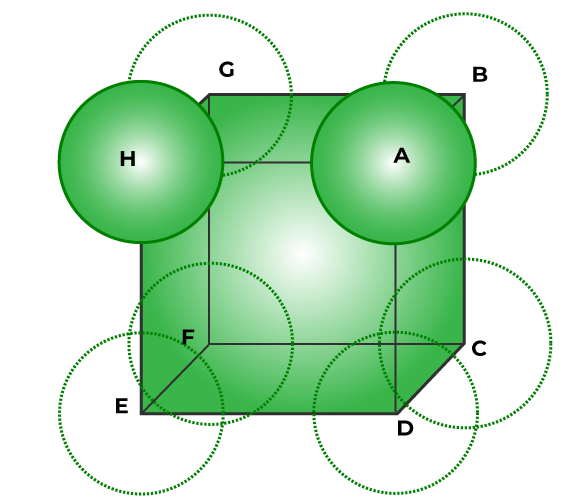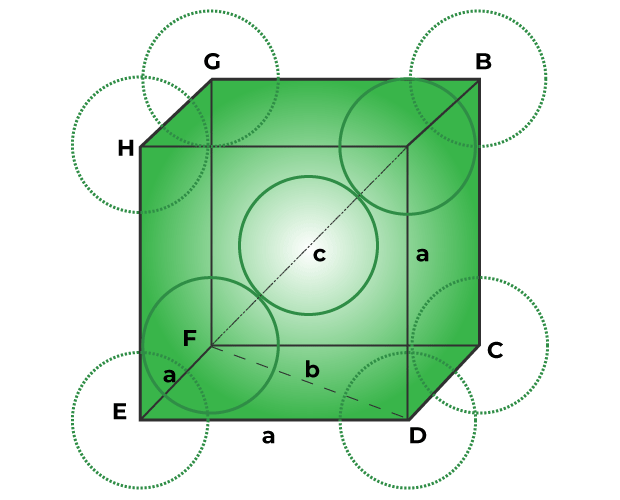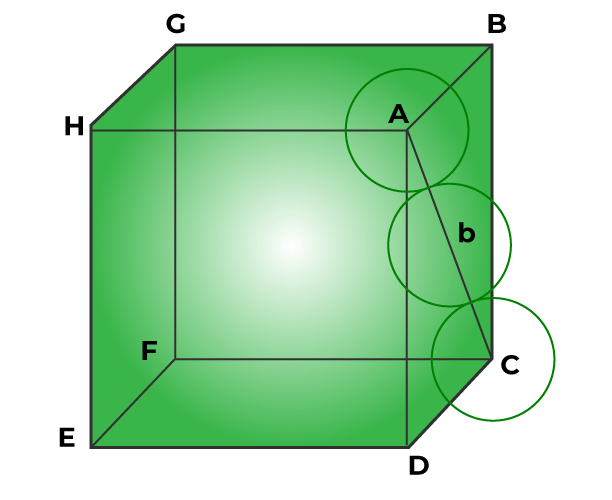GFG App
Open AppBrowser
Continue

A crystal lattice is made up of a relatively large number of unit cells, each of which contains one constituent particle at each lattice point. A three-dimensional structure with one or more atoms can be thought of as the unit cell. Regardless of the packing method, there are always some empty spaces in the unit cell. The packing fraction of the unit cell is the percentage of empty spaces in the unit cell that is filled with particles. In this article, we shall learn about packing efficiency.

## Packing Efficiency

Packing efficiency is the fraction of a solid’s total volume that is occupied by spherical atoms.

Packing Efficiency is the proportion of a unit cell’s total volume that is occupied by the atoms, ions, or molecules that make up the lattice. It is the entire area that each of these particles takes up in three dimensions. It can be understood simply as the defined percentage of a solid’s total volume that is inhabited by spherical atoms.

Similar to the coordination number, the packing efficiency’s magnitude indicates how tightly particles are packed.

## Packing Efficiency Formula

The formula for effective packing is,

Packing efficiency = (Volume occupied by particles in unit cell / Total volume of unit cell) × 100

### Packing Efficiency Determining Factors

The following elements affect how efficiently a unit cell is packed:

1. The number of atoms in lattice structure’s
2. Unit cell volume
3. Atoms volume

Packing Efficiency can be evaluated through three different structures of geometry which are:

1. Simple Cubic Lattice
2. Body-Centered Cubic Lattice
3. Face-Centered Cubic Lattice (or CCP or HCP Lattice)

## Packing Efficiency of Metal Crystal in Simple Cubic Lattice

The steps below are used to achieve Simple Cubic Lattice’s Packing Efficiency of Metal Crystal:

In a simple cubic unit cell, spheres or particles are at the corners and touch along the edge. Below is an diagram of the face of a simple cubic unit cell.It is evident that,

a=2r or r = a/2  …(Equation 1)

Where, r is the radius of atom and a is the length of unit cell edge.

Step 2: Volume of sphere:

Volume of a sphere = (4/3π)(r3)

Substitution for r from equation 1 gives,

∴ Volume of one particle = (4/3π)(a/2)3

∴ Volume of one particle = πa3 / 6  …(Equation 2)

Step 3: Total volume of particles:

Simple cubic unit cells only contain one particle.

∴ Volume occupied by particle in unit cell = πa3 / 6

Step 4: Packing Efficiency:

We have,

Packing efficiency = (Volume occupied by particles in unit cell / Total volume of unit cell) × 100

∴ Packing efficiency = ((πa3 / 6) / a3) × 100

∴ Packing efficiency = 100π / 6

∴ Packing efficiency = (100 × 3.142) / 6

∴ Packing efficiency = 52.36 %

Therefore, in a simple cubic lattice, particles take up 52.36 % of space whereas void volume, or the remaining 47.64 %, is empty space.

## Packing Efficiency of Metal Crystal in Body-centered Cubic Lattice

The steps below are used to achieve Body-centered Cubic Lattice’s Packing Efficiency of Metal Crystal

The corners of the bcc unit cell are filled with particles, and one particle also sits in the cube’s middle. The cube’s center particle hits two corner particles along its diagonal, as seen in the figure below.The Pythagorean theorem is used to determine the particle’s (sphere’s) radius.

In triangle EFD,

b2 = a2 + a2

∴ b2 = 2a2

∴ b = √2 a

According to the Pythagoras theorem, now in triangle AFD,

c2 = a2 + b2

∴ c2 = a2 + 2a2

∴ c2 = 3a2

∴ c = √3 a

We can rewrite the equation as since the radius of each sphere equals r.

c = 4r

√3 a = 4r

∴ r = √3/4 a  …(Equation 1)

Step 2: Volume of sphere:

Volume of sphere particle = 4/3 πr3. Substitution for r from equation 1, we get

∴ Volume of one particle = 4/3 π(√3/4 a)3

∴ Volume of one particle = 4/3 π × (√3)3/64 × a3

∴ Volume of one particle = √3 πa3 / 16

Step 3: Total volume of particles:

Unit cell bcc contains 2 particles. Hence,

volume occupied by particles in bcc unit cell = 2 × ((2√3 πa3) / 16)

∴ volume occupied by particles in bcc unit cell = √3 πa3 / 8  …(Equation 2)

Step 4: Packing Efficiency:

We have,

Packing efficiency = (Volume occupied by particles in unit cell / Total volume of unit cell) × 100

∴ Packing efficiency = (√3 πa3 / 8a3) × 100

∴ Packing efficiency = 68 %

As a result, atoms occupy 68 % volume of the bcc unit lattice while void space, or 32 %, is left unoccupied.

## Packing Efficiency of Metal Crystal in Face-centered Cubic Lattice

The steps below are used to achieve Face-centered Cubic Lattice’s Packing Efficiency of Metal Crystal:

The corner particles are expected to touch the face ABCD’s central particle, as indicated in the figure below.According to Pythagoras Theorem, the triangle ABC has a right angle.

AC2 = AB2 + BC2

∴ AC2 = a2 + a2

∴ AC2 = 2a2

∴ AC = √2 a  …(Equation 1)

We can rewrite the equation as since the radius of each sphere equals r.

AC = 4r  …(Equation 2)

From equation 1 and 2, we get

√2 a = 4r

∴ r = a / 2√2  …(Equation 3)

Step 2: Volume of sphere:

Volume of sphere particle = 4/3 πr3. Substitution for r from equation 3, we get

∴ Volume of one particle = 4/3 π(a / 2√2)3

∴ Volume of one particle = 4/3 πa3 × (1/2√2)3

∴ Volume of one particle = πa3 / 12√2

Step 3: Total volume of particles:

Unit cell bcc contains 4 particles. Hence,

volume occupied by particles in FCC unit cell =  4 × πa3 / 12√2

∴ volume occupied by particles in FCC unit cell = πa3 / 3√2

Step 4: Packing Efficiency:

We have,

Packing efficiency = (Volume occupied by particles in unit cell / Total volume of unit cell) × 100

∴ Packing efficiency = πa3 / 3√2 a3 × 100

∴ Packing efficiency = 74 %

As a result, particles occupy 74% of the entire volume in the FCC, CCP, and HCP crystal lattice, whereas void volume, or empty space, makes up 26% of the total volume.

### Importance of Efficient Packing

Packing Efficiency is important as:

1. The object’s sturdy construction is shown through packing efficiency.
2. It shows various solid qualities, including isotropy, consistency, and density.
3. Numerous characteristics of solid structures can be obtained with the aid of packing efficiency.

## Solved Examples of Packing Efficiency

Example 1: Calculate the total volume of particles in the BCC lattice.

Solution:

Unit cell bcc contains 2 particles. Hence,

volume occupied by particles in bcc unit cell = 2 × ((2√3 πa3) / 16)

∴ volume occupied by particles in bcc unit cell = √3 πa3 / 8

Example 2: Calculate Packing Efficiency of Face-centered cubic lattice.

Solution:

We have,

Packing efficiency = (Volume occupied by particles in unit cell / Total volume of unit cell) × 100

∴ Packing efficiency = πa3 / 3√2 a3 × 100

∴ Packing efficiency = 74 %

Example 3: Calculate Packing Efficiency of Simple cubic lattice.

Solution:

We have,

Packing efficiency = (Volume occupied by particles in unit cell / Total volume of unit cell) × 100

∴ Packing efficiency = ((πa3 / 6) / a3) × 100

∴ Packing efficiency = 100π / 6

∴ Packing efficiency = (100 × 3.142) / 6

∴ Packing efficiency = 52.36 %

Example 4: Calculate the volume of spherical particles of the body-centered cubic lattice.

Solution:

Volume of sphere particle = 4/3 πr3. Substitution for r from  r = √3/4 a, we get

∴ Volume of one particle = 4/3 π(√3/4 a)3

∴ Volume of one particle = 4/3 π × (√3)3/64 × a3

∴ Volume of one particle = √3 πa3 / 16

## FAQs on Packing Efficiency

Question 1: What is Face Centered Unit Cell?

In a face centered unit cell the corner atoms are shared by 8 unit cells. Additionally, it has a single atom in the middle of each face of the cubic lattice. Two unit cells share these atoms in the faces of the molecules.

Question 2: What role does packing efficiency play?

Roles of Packing efficiency:

1. The structure of the solid can be identified and determined using packing efficiency.
2. Consistency, density, and isotropy are some of the effects. How well an element is bound can be learned from packing efficiency.
3. Chemical, physical, and mechanical qualities, as well as a number of other attributes, are revealed by packing efficiency.

Question 3: How effective are SCC, BCC, and FCC at packing?

The packing efficiency of different solid structures is as follows.

1. Simple Cubic (SCC) – 52.4%
2. Body Centered (BCC) – 68%
3. Face Centered (FCC) – 74%

Question 4: How effective is packing?

Packing efficiency is the proportion of a given packing’s total volume that its particles occupy. In the crystal lattice, the constituent particles, such as atoms, ions, or molecules, are tightly packed. They have two options for doing so: cubic close packing (CCP) and hexagonal close packing (HCP). The complete amount of space is not occupied in either of the scenarios, leaving a number of empty spaces or voids.

Question 5: What are the factors of packing efficiency?